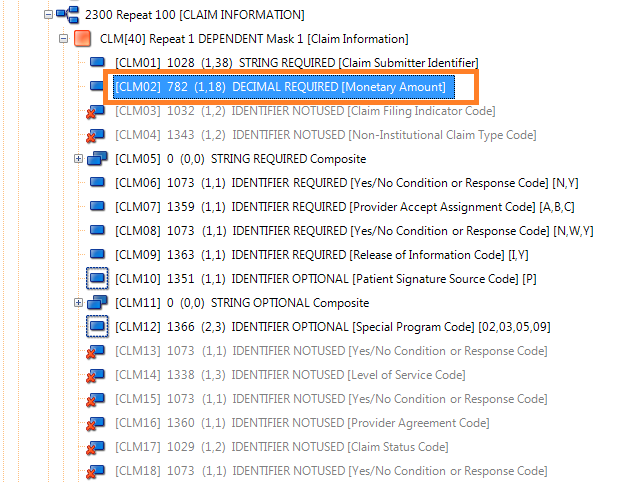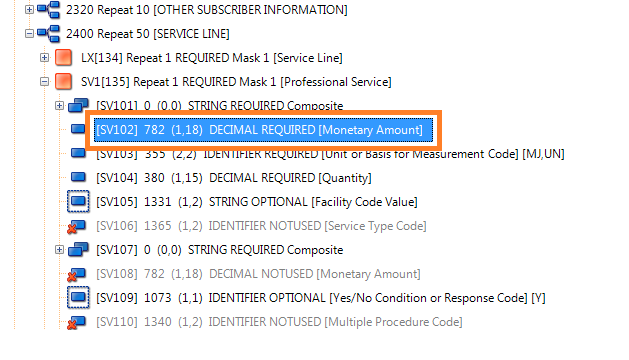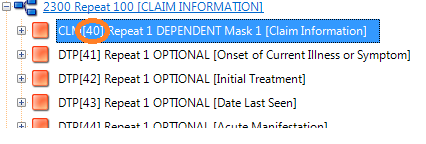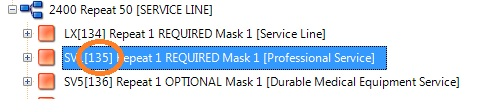In this tutorial we’ll discuss how to do Summary Balancing validation on EDI data.

In some EDI transactions there is a top level segment that contains an element whose value represents the total amount of some kind.  For example, in an 837 Professional Health care claim transaction there is a CLM segment whose second element (CLM02) contains the total amount charged for the claim.The CLM02 below represents the total amount for a claim (\$80)

`CLM*1029353-03*80***11<B<1*Y*A*Y*Y~`

So where does this total amount come from?  It’s nothing but the sum of each repetition of the SV1 segment’s element two (SV102)This makes sense in an 837 Professional Health care claim transaction.  For example, if you have 4 professional visits (4 repetition of loop 2400, each with an SV1 segment) and each cost \$20 then the claim total (CLM02) should be \$80.  An example of one  SV1 segment is

`SV1*HC<97530*20*UN*3***1<2~`

Here is a full excerpt from a sample EDI file.  Note that there are 4 SV1 segments whose element SV102 each has a value of \$20

```CLM*1029353-03*80***11<B<1*Y*A*Y*Y~
HI*BK<6268*BF<78930~
NM1*DN*1*SMITH*BOBBY****XX*123456789~
REF*1G*T32418~
NM1*82*2*SMITH*****XX*123456789~
REF*G2*00228498B~
NM1*77*2*SMITH LANEY*****XX*123456789~
N3*1111 DRICE PLACE~
N4*SOMEWHERE*NY*11119~
LX*1~
SV1*HC<87086*20*UN*1***1~
DTP*472*D8*20070118~
LX*2~
SV1*HC<97530*20*UN*3***1<2~
DTP*472*D8*20070118~
LX*3~
SV1*HC<97530*20*UN*3***1<2~
DTP*472*D8*20070118~
LX*4~
SV1*HC<97530*20*UN*3***1<2~
DTP*472*D8*20070118~```

Having a total amount field lets systems know the total amount charged for each claim.

What would happen in the above example if CLM02 value was \$90?  That would not fully represent the total value charged for the claim.  This can result in other errors while processing the claim.  You would surely want to know when the totals are off.

The EDIValidator supports summary balance checking to make sure that totals are correct.  To configure it all we need is the Segment Ordinals of the segment and elements that we are trying to verify.

The Segment Ordinal of the CLM segment is displayed at the beginning of the segment in EDI Rules Creator Studio.

For CLM it’s 40And for SV1 it’s 135In our 5010 Validation Rules file we just need to add a totals check

```SUMMARY
40:2=135:2```

This means that CLM element 2 (40:2) must contain the total of each SV1 element 2 (135:2).  If this is not true an error will be generated.

By default we are taking the sum of each SV1 element 2 (135:2).  However, summaries also support differences as well.

For example, in the 5010 835 Implementation Guide element BPR02 has to contain the sum of the element values of CAS04 subtract the total of PLB04 + PLB06 + PLB08 + PLB10 + PLB12 + PLB14.  Essentially,

BPR02 = SUM(CAS04) – (PLB04+PLB06+PLB06+PLB08+PLB10+PLB12+PLB18)

In this case a summary can be written as

```SUMMARY
4:2=25:4,-53:4,-53:6,-53:8,-53:10,-53:12,-53:14```

Now that was easy!

Take Charge Of EDI

## RDPCrystal EDI Library